# A Simple Method for Closure of Triangular Skin Defects

## Summary

This article describes a simple method for closure of triangular skin defects and also makes a comparison with other incisions that can be used for the same purpose.

## Introduction

Most of the times, skin lesions have a round shape but in certain cases the lesions could present themselves as a triangular shape. In the recent medical literature there are a few methods that can be used for closure of triangular skin defects such as the L-shaped flap for triangular skin defects of Sakai and Soeda , the Mutaf triangular closure  and the triangular excision with primary closure for small lesions of Amaral Filho and colleagues . The tracing of these incisions is rather complicated and the resultant flaps have a narrow base which could compromise their survival. In this article I am presenting a simple method for closure of triangular skin defects that is easy to trace and to memorize. In addition, the bases of the flaps are relatively wide which will minimize the risk of necrosis and at the same time will cover the central portion of the skin defect.

## Description of the Method

A triangular incision is traced around the skin lesion and a smaller triangular extension is demarcated on either side of the triangular excision [Figure 1]. The bases of the excision triangle and the extension triangle have the same length where their length (b and b’) is equivalent to ¾ the whole base (d) of the excision triangle. The resultant defect shows two triangles connected at their base and also two wide base flaps A and B with obtuse angles of 120 and 135 degrees respectively. These flaps can be mobilized in opposite directions resulting in a suture line with a discrete “S” shape that measures 7 cm in length only. After closure of the incision the flaps show no distortion of the minimal tension lines of the skin. In case that the skin lesion has the shape of an acute isosceles triangle, a similar shape excision triangle [Figure 2] can be used but the extension triangle keeps the same proportion as in the equilateral excision triangle. In this case the resultant flaps (A and B) have, also, a wide base with obtuse angles of 113 and 135 degrees respectively. The resultant suture line is similar to the previous incision and measures 8 cm in length only.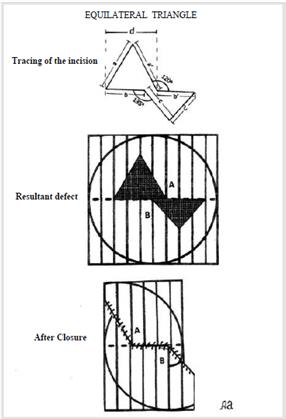Figure 1: Shows the tracing of an Equilateral Triangular Incision and a smaller triangular extension so, the resultant defect consists of two triangles connected at their base. The suture line shows a discrete “S” shape with no distortion of the minimal tension lines of the skin, and its length is 7 cm only. The bases of the flaps A and B are quite wide which minimize the possibility of ischemia or necrosis.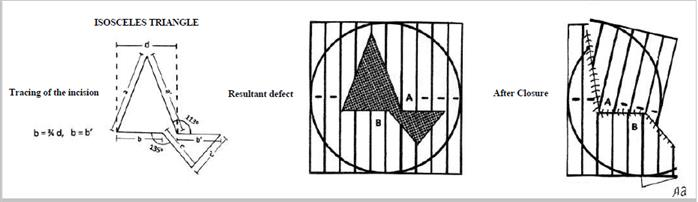Figure 2: Shows the tracing of an isosceles triangle with a resultant defect and a suture line after closure. The flaps A and B have also wide bases and the suture line has a mild “S” shape with slight distortion of the minimal tension lines of the skin. The length of the suture line is 8 cm only.

For practical purposes, a working model of bond paper can be made to be used later on the spot in order to evaluate the different possibilities according with the anatomy and the particular skin conditions surrounding the lesion to be removed. This working model can be enlarged or reduced in size as needed by using a regular copying machine.

## Comparison with other Incisions

The rhombic incision [Figure 3] is simple to trace and the resultant suture line is straight and with negligible distortion of the minimal tension lines of the skin and measures 7 cm in length only.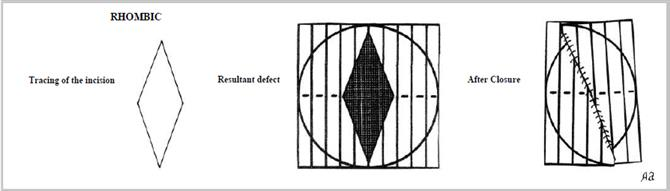Figure 3: Shows the tracing of a rhombic incision with a resultant defect and a suture 6 line after closure with no distortion of the minimal tension lines of the skin. The length of the suture line is 7 cm only.

The ellipsoid incision [Figure 4] is a rounded version of the rhombic incision and produces a mild “S” shape suture line with negligible distortion on the minimal tension lines and mesures 9.5 cm in length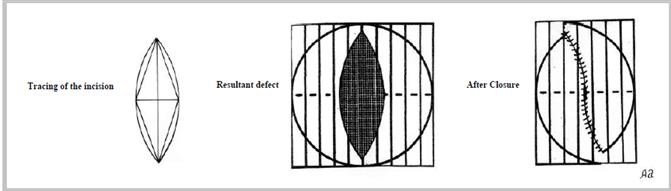Figure 4: Shows the tracing of an ellipsoid incision with a resultant defect and a suture line after closure, similar to the rhombic incision. The length of the suture line is 9.5 cm only.

The Sakai-Soeda L-shaped flap [Figure 5] shows a small sharp pointed flap (A) of 45 degrees that requires minimal dissection but produces a suture line with a complicated triangular gap that could prove difficult to close. The resultant suture line measures 19 cm in length and the minimal tension lines show marked distortion.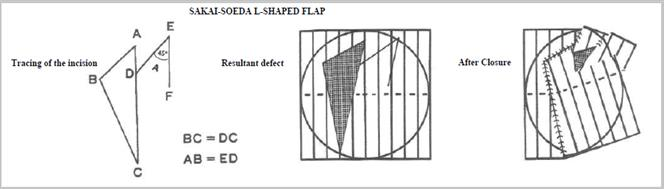Figure 5: Shows the tracing of the Sakai-Soeda L-shaped Flap with a small sharp flap of 45 degrees and a long suture line. The closure of the suture line presents a significant problem due to a triangular gap at the upper end of the incision. The length of the suture line is 19 cm which is almost three times than the length of the suture line of the new triangular incisions presented here. Besides this, the minimal tension lines of the skin are quite distorted.

The Mutaf triangular closure [Figure 6] produces a long sharp pointed flap of 45 degrees that could compromise its blood supply. Besides this, the mobilization of this flap requires an extensive dissection far away from the primary lesion which could result in excessive bleeding. The resultant suture line measures 20.3 cm in length and produces large gaps that could be difficult to close. In addition the minimal tension lines are quite distorted. The Half Moon and the Goblet incisions [Figure 7] described in my previous article  have significant advantages over the previous incisions cited here. They are easy to memorize and produce two wide flaps (A and B) which will minimize the risk of necrosis. Besides this, the mobilization of the flaps requires moderate dissection and the waste of the skin is minimal if the whole traced area is removed (21% and 19%) respectively. The resultant suture lines show no significant distortion of the minimal tension lines. The length of the suture line is 13 cm for the Half Moon incision and 13.5 cm for the Goblet incision. Both incisions can be used when the skin lesions have the shape of an obtuse isosceles triangle.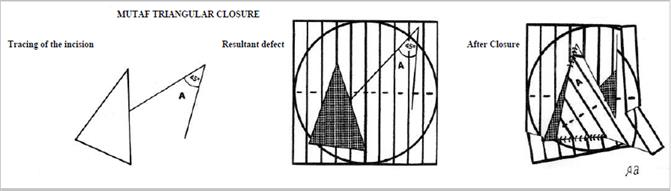Figure 6: Shows the tracing of the Mutaf Triangular Closure with the resulting defect and suture line after closure. The flap A is long and narrow which could compromise its survival, and the suture line shows two large triangular gaps that could prove difficult to close. The length of the suture line is 20.3 cm which is almost three times more than the new triangular incisions presented here. In addition, the minimal tension lines are quite distorted.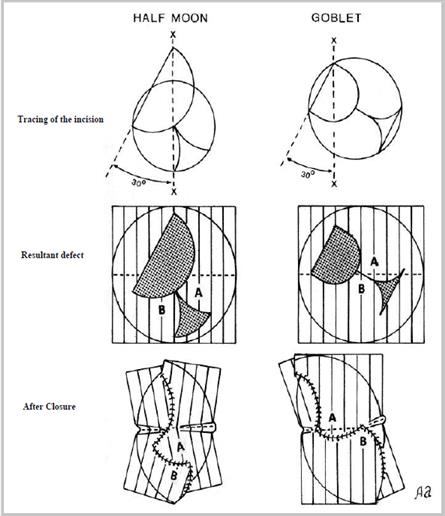Figure 7: Shows the tracing of the Half Moon and Goblet incisions with their resultant defect and the suture lines after closure. Please note that the Goblet incision shows negligible distortion of the minimal tension lines of the skin. The length of the suture line is 13 cm for the Half Moon incision, and 13.5 cm for the Goblet incision.

## Geometrical Analysis

An analysis of the triangular incisions described here is done for comparison with other incisions that could be used to close triangular defects. In this analysis a circular defect of 3 cm in diameter is used as a common denominator and in this manner we can obtain a relative waste for each incision.

The Equilateral Triangular Incision ABCD [Figure 8] measures 10.75 cm2 (5 ×4.3 ÷ 2) with an intrinsic waste is 3.68 cm2 (10.75 − 7.07). The triangular extension E measures 6.87 cm2 (5 ×2.75 ÷ 2) which represents the extrinsic waste. If we add these two values we obtain a total waste of 10.55 cm2 and a relative waste of 149% (10.55 ÷ 7.07) if the circle A is removed, but if the whole triangle is removed, the total waste is 53.5% only.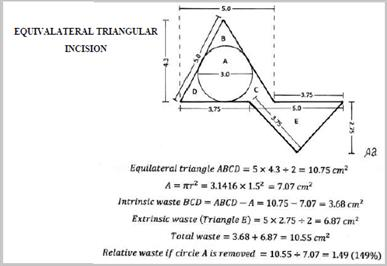Figure 8: Shows a geometric analysis of an Equilateral Triangular Incision where the relative waste of the skin is 149% (10.55 ÷ 7.07) if circle A is removed. This compares favorably with the relative waste of the elliptical incision that is 171%. But if the whole triangle ABCD is removed, the total waste is 63.9% only.

The Isosceles Triangular Incision ABCD [Figure 9] measures 12.9 cm2 (4.3 X 6.0 ÷ 2) with an intrinsic waste of 5.83 cm2 (12.9 − 7.07). The triangular extension E measures 4.94 cm2 (4.3 X 2.3 ÷ 2) which represents the extrinsic waste. If we add these two values we obtain a total waste of 10.77 cm2 and a relative waste of 152% (10.77 ÷ 7.07) if the circle A is removed, but if the whole triangle ABCD is removed the total waste is 63.9% only.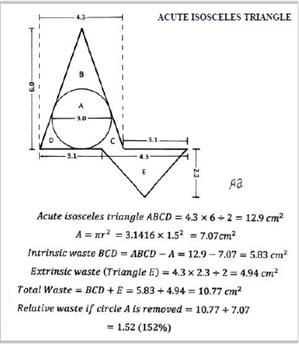Figure 9: shows a geometric analysis of an Acute Isosceles Triangular incision where the 7 relative waste of the skin is 152% (10.77 ÷ 7.07) if circle A is removed. But, if the whole triangle ABCD is removed, the total waste is 38.2% only.

The Rhombus Incision ABC [Figure 10] measures 14.40 cm2 (3.2 X 9.0 ÷ 2) with an intrinsic waste BC of 7.33 cm2 (14.40-7.07) so its relative waste is 103% (7.33 ÷ 7.07) if the circle A is removed. But if the triangle B + ½ A is removed the total waste is 100%.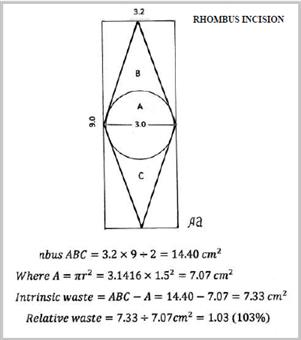Figure 10: shows a geometric analysis of a rhombic incision where the relative waste of the skin is 103% (7.33 ÷ 7.07) if circle A is removed. But, if the triangle B + ½A is removed the total waste is 100%.

The Ellipsoid Incision [Figure 11] is calculated using a sector GDE of 40 degrees in a circle of 7 cm radius. The segment “a” is obtained by subtracting the triangle GEF from the sector GDF which is equal to 1.19 cm2 and the sum of segments “abcd” would be 4.77 cm2. The intrinsic waste is the sum of 7.33 (the rhombus intrinsic waste) plus 4.77 which is equal to 12.10 cm2, and the relative waste is 171% (12.10 ÷ 7.07) if the circle A is removed, but if the triangle B + ½ A is removed the total waste is 100%.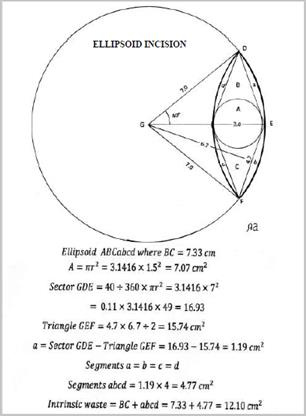Figure 11: Shows a geometric analysis of an Acute Isosceles Triangular incision where the relative waste of the skin is 152% (10.77 ÷ 7.07) if circle A is removed the total waste is 100%.

The Sakai-Soeda L-shaped Flap [Figure 12] has a resected area of 16.8 cm2 with an intrinsic waste of 9.73 cm2 (16.8 – 7.07) and a relative waste of 137% (9.73 ÷ 7.07) if the circle A is removed, but if the whole triangle ABC is removed the total waste is 0%.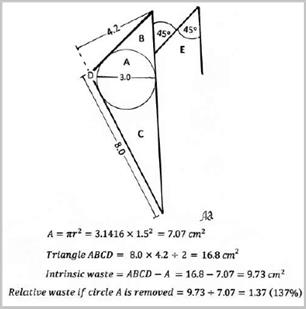Figure 12: Shows a geometric analysis of the Sakai-Soeda L-shaped flap where the relative waste of the skin is 137% (9.73 ÷ 7.07) if circle A is removed. But if the whole triangle ABC is removed the total waste is 0%. On the other hand, the length of the suture line is 19 cm which is almost three times the length of Equilateral Triangular incision.

The Mutaf Triangular Closure [Figure 13] has a resected area of 12.60 cm2 with an intrinsic waste of 5.53 cm2 ( 12.60 – 7.07) and a relative waste of 78% (5.53 ÷ 7.07) if the circle A is removed, but if the whole triangle ABCD is removed the total waste is 0%.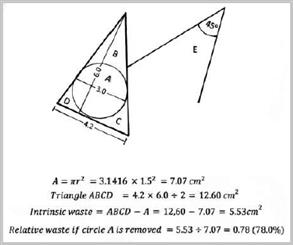Figure 13: shows the geometric analysis of the Mutaf Triangular Closure where the relative waste of the skin is 78% (5.53 ÷ 7.07) if circle A is removed. But if the whole triangle ABCD is removed the total waste is 0%. On the other hand, the length of the suture line is 20.3 cm which is three times the length of the Equilateral Triangular Incision.

The Half Moon Incision [Figure 14] has an intrinsic waste BC of 7.07 cm2 (14.14 -7.07) and an extrinsic waste (D) of 3.07 cm2 so the total waste is 10.14 cm2 which represents a relative waste of 143% (10 .14 ÷ 7.07) if circle A is removed. Now, if the triangle EFG is removed the total waste is 8.20 cm2 (5.13 + 3.07) which represents a relative waste of 91%. But, if the whole traced area is removed the relative waste is 21% (3.14 ÷ 14.14) only.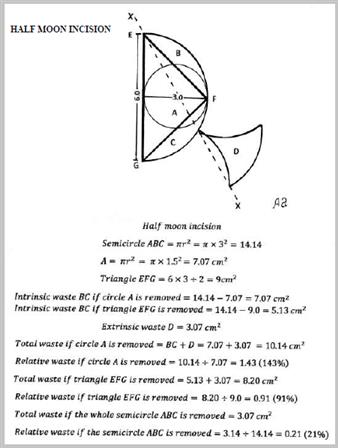Figure 14: shows the geometric analysis of the Half-moon incision where the relative waste of the skin is 143% (10.14 ÷ 7.07) if circle A is removed. It also shows a relative waste of 91% (8.20 ÷ 9.0) if the obtuse isosceles triangle EFG is removed. If the whole semicircle ABC is removed,the relative waste of the skin is 21% (3.14 ÷ 14.14) only. The length of the suture line is 13 cm so the number of sutures required to close the wound is considerably reduced.

The Goblet Incision [Figure 15] has an intrinsic waste BC of 4.25 2 (11.32-7.07) and an extrinsic waste (D) of 2.18 cm2 so the total waste is 6.43 cm2 which represents a relative waste of 90% (6.43 ÷ 7.07) if circle A is removed. Now, if the triangle EFG is removed the total waste is 6.75 cm2 (4.57 + 2.18) which represents a relative waste of 147% (6.75 ÷ 4.57). But, if the whole traced area is removed the relative waste is 19% (2.18 ÷ 11.32) only.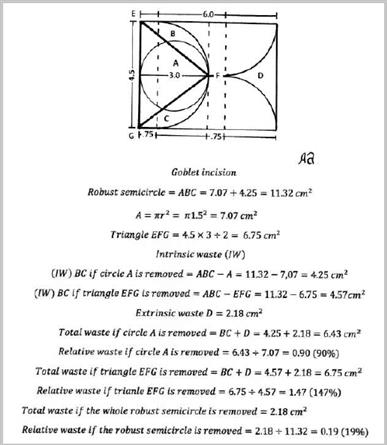Figure 15: Shows the geometric analysis of the Goblet incision where the relative waste of the skin is 90% (6.43 ÷ 7.07) if circle A is removed. It also shows a relative waste of 147% (6.75 ÷ 4.57)if the obtuse isosceles triangle EFG is removed. If the whole robust semicircle ABC isr emoved, the relative waste of the skin is 19% (2.18 ÷ 11.32) only. On the other hand, the suture line length is 13.5 cm which is twice the length of the new triangular incisions described here.

## Discussion

We can use a variety of incisions to close triangular skin defects when the skin lesions have a triangular shape. These situations could happen when closing large meningomyeloceles [5-7], or when resecting pilonidal sinuses  or when removing triangular defects of the infraorbital region . The Triangular Incisions presented here are easy to trace and to memorize, and more important, they have wide base flaps which will minimize the risk of ischemia or necrosis. Furthermore, the resultant suture lines are away from the central area of the incision and the edges of the suture line complies with the principle of reciprocity so the approximation of the edges ends up even thus avoiding the formation of wide gaps that could be very difficult to close. In addition, the Triangular incisions presented here have the advantage of producing a short suture line in relation to the Sakai-Soeda L-shaped Flap and the Mutaf Triangular Closure which will reduce significantly the number of sutures required to close the wound. In conclusion, each incision has its own advantages and disadvantages depending on the local anatomy and the skin conditions so the surgeon should decide which incision is more appropriate.

## References

1. Sakai S and Soeda S. L-shaped flap for triangular skin defects. Br J Plast Surg. 1988; 41: 628-632. [Crossref]
2. Mutaf M, Bekerecioglu M, Erkutlu I and Bulut O. A new technique for closure of large meningomyocele defects. Ann Plast Surg. 2007; 59:538-543. [Crossref]
3. Filho EA, Wojcik AS, Brenner FA, Jesus Rodriguez Santamaria and Betina Werner. Cutaneous triangular excision with primary closure. Surg Cosmet Dermatol. 2011; 3: 31-35. [Crossref]
4. Alvarado A. Designing flaps for closure of circular and semicircular skin defects. Plastic Reconstr Surg Glob Open. 2016; 4: e607. [Crossref]
5. Cole P, Armenta A, Dauser R and Larry Hollier Jr. Simplifying soft tissue closure of large meningomyelocle defect: a technical note. J Plast & Reconstr Surg. 2008; 6: 192-194. [Crossref]
6. Gumus N. A new approach to closure of myelomeningocele defect: Z advancement-rotation flap. Ann Plast Surg. 2008; 61: 640-645. [Crossref]
7. Zobaci E, Temel M, Yasti AC, Musa Zorlu, Faruk Coskun, Mete Dolapci, et al. The use of the triangular closure technique for defect coverage in pilonidal sinus treatment. Int J Clin Exp Surg. 2016; 9: 12804- 12810. [Crossref]
8. Cecchi R, Bartoli R, Brunetti L and Giovanni Troiano. Mutaf triangular flap technique in the repair of infraorbital skin losses: Report of 5 cases. J Cutan Aesthet Surg. 2014; 7: 176- 177. [Crossref]

#### Editorial Information

Editor-in-Chief

Article Type

Research Article

Publication history

Accepted date: June 12, 2017

Published date: June 14, 2017

© 2017 Alfredo Alvarado, This is an open-access article distributed under the terms of the Creative Commons Attribution License, which permits unrestricted use, distribution, and reproduction in any medium, provided the original author and source are credited.

Citation

Alfredo Alvarado. A Simple Method for Closure of Triangular Skin Defects. Adv Plast Reconstr Surg. 2017; 1(1):100-107.

#### Corresponding Author

Independent Researcher, Calle 25B #74B-19, Apto. 201, Bogota 1101, Colombia, E-mail: alfredoalvara@hotmail.com

#### Figures and DataFigure 1: Shows the tracing of an Equilateral Triangular Incision and a smaller triangular extension so, the resultant defect consists of two triangles connected at their base. The suture line shows a discrete “S” shape with no distortion of the minimal tension lines of the skin, and its length is 7 cm only. The bases of the flaps A and B are quite wide which minimize the possibility of ischemia or necrosis.Figure 2: Shows the tracing of an isosceles triangle with a resultant defect and a suture line after closure. The flaps A and B have also wide bases and the suture line has a mild “S” shape with slight distortion of the minimal tension lines of the skin. The length of the suture line is 8 cm only.Figure 3: Shows the tracing of a rhombic incision with a resultant defect and a suture 6 line after closure with no distortion of the minimal tension lines of the skin. The length of the suture line is 7 cm only.Figure 4: Shows the tracing of an ellipsoid incision with a resultant defect and a suture line after closure, similar to the rhombic incision. The length of the suture line is 9.5 cm only.Figure 5: Shows the tracing of the Sakai-Soeda L-shaped Flap with a small sharp flap of 45 degrees and a long suture line. The closure of the suture line presents a significant problem due to a triangular gap at the upper end of the incision. The length of the suture line is 19 cm which is almost three times than the length of the suture line of the new triangular incisions presented here. Besides this, the minimal tension lines of the skin are quite distorted.Figure 6: Shows the tracing of the Mutaf Triangular Closure with the resulting defect and suture line after closure. The flap A is long and narrow which could compromise its survival, and the suture line shows two large triangular gaps that could prove difficult to close. The length of the suture line is 20.3 cm which is almost three times more than the new triangular incisions presented here. In addition, the minimal tension lines are quite distorted.Figure 7: Shows the tracing of the Half Moon and Goblet incisions with their resultant defect and the suture lines after closure. Please note that the Goblet incision shows negligible distortion of the minimal tension lines of the skin. The length of the suture line is 13 cm for the Half Moon incision, and 13.5 cm for the Goblet incision.Figure 8: Shows a geometric analysis of an Equilateral Triangular Incision where the relative waste of the skin is 149% (10.55 ÷ 7.07) if circle A is removed. This compares favorably with the relative waste of the elliptical incision that is 171%. But if the whole triangle ABCD is removed, the total waste is 63.9% only.Figure 9: shows a geometric analysis of an Acute Isosceles Triangular incision where the 7 relative waste of the skin is 152% (10.77 ÷ 7.07) if circle A is removed. But, if the whole triangle ABCD is removed, the total waste is 38.2% only.Figure 10: shows a geometric analysis of a rhombic incision where the relative waste of the skin is 103% (7.33 ÷ 7.07) if circle A is removed. But, if the triangle B + ½A is removed the total waste is 100%.Figure 11: Shows a geometric analysis of an Acute Isosceles Triangular incision where the relative waste of the skin is 152% (10.77 ÷ 7.07) if circle A is removed the total waste is 100%.Figure 12: Shows a geometric analysis of the Sakai-Soeda L-shaped flap where the relative waste of the skin is 137% (9.73 ÷ 7.07) if circle A is removed. But if the whole triangle ABC is removed the total waste is 0%. On the other hand, the length of the suture line is 19 cm which is almost three times the length of Equilateral Triangular incision.Figure 13: shows the geometric analysis of the Mutaf Triangular Closure where the relative waste of the skin is 78% (5.53 ÷ 7.07) if circle A is removed. But if the whole triangle ABCD is removed the total waste is 0%. On the other hand, the length of the suture line is 20.3 cm which is three times the length of the Equilateral Triangular Incision.Figure 14: shows the geometric analysis of the Half-moon incision where the relative waste of the skin is 143% (10.14 ÷ 7.07) if circle A is removed. It also shows a relative waste of 91% (8.20 ÷ 9.0) if the obtuse isosceles triangle EFG is removed. If the whole semicircle ABC is removed,the relative waste of the skin is 21% (3.14 ÷ 14.14) only. The length of the suture line is 13 cm so the number of sutures required to close the wound is considerably reduced.Figure 15: Shows the geometric analysis of the Goblet incision where the relative waste of the skin is 90% (6.43 ÷ 7.07) if circle A is removed. It also shows a relative waste of 147% (6.75 ÷ 4.57)if the obtuse isosceles triangle EFG is removed. If the whole robust semicircle ABC isr emoved, the relative waste of the skin is 19% (2.18 ÷ 11.32) only. On the other hand, the suture line length is 13.5 cm which is twice the length of the new triangular incisions described here.